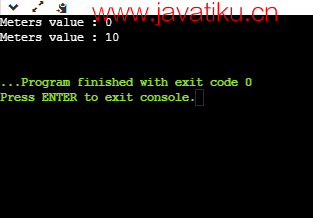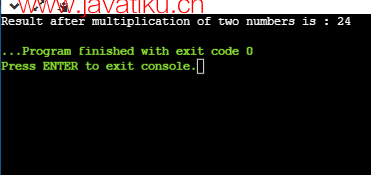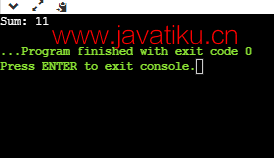# C++教程-C++中的友元函数## C++中的友元函数

### 友元函数的特点：

1. 友元函数不在其被声明为友元的类的作用域内。
2. 不能使用对象调用友元函数，因为它不在该类的作用域内。
3. 可以像普通函数一样调用友元函数，无需使用对象。
4. 不能直接访问成员名称，而必须使用对象名和成员名的点成员运算符。
5. 友元函数可以在类的私有或公有部分声明。

### 为什么我们需要在C++中使用友元函数？

• 在C++中，友元函数用于直接访问类的私有数据，而不使用该类的对象。
• 友元函数还用于执行运算符重载。就像我们已经了解到的函数重载一样，运算符也可以通过运算符重载进行重载。

1. 友元函数使用friend关键字声明。
2. 它不是类的成员，但是它是类的友元。
3. 由于它不是类的成员，因此可以像普通函数一样定义。
4. 友元函数不能直接访问类的数据成员，而是将一个对象作为参数传递。
5. 它类似于普通函数。
6. 如果我们想在函数中共享多个类的数据，则可以使用友元函数。

``````class class_name
{
friend data_type function_name(argument/s);            // 友元函数的语法
};      ``````

``````#include <iostream>
using namespace std;
class Distance
{
private:
int meters;
public:
// 构造函数
Distance()
{
meters = 0;
}
// display_data() 方法的定义
void display_data()
{
std::cout << "Meters value : " << meters<<std::endl;
}

// 友元函数的原型。

};

// 友元函数的定义
{
d.meters = d.meters+10; // 将meters的值增加10
}

// main() 方法
int main()
{
Distance d1; // 创建Distance类的对象
d1.display_data(); // meters = 0
d1.display_data(); // meters = 10
return 0;
}  ````````````// 使用友元函数将两个不同类的成员相加
#include <iostream>
using namespace std;
// 类的前向声明
class ClassB;

// 类的声明
class ClassA {
public:
// 构造函数 ClassA() 将 num1 初始化为 12
ClassA()
{
num1 = 12;

}

private:
int num1;  // 整数变量的声明

// 友元函数声明
friend int multiply(ClassA, ClassB);
};
class ClassB {
public:
// 构造函数 ClassB() 将 num2 初始化为 2
ClassB()
{
num2 = 2;
}
private:
int num2;  // 整数变量的声明
// 友元函数声明
friend int multiply(ClassA, ClassB);
};

// 访问两个类的成员
int multiply(ClassA object1, ClassB object2)
{
return (object1.num1 * object2.num2);
}

int main() {
ClassA object1;   // ClassA对象的声明
ClassB object2;   // ClassB对象的声明
cout << "两个数相乘的结果为: " << multiply(object1, object2);
return 0;
}``````### 友元类在C++中

``````class Class1;
class Class2
{
// Class1是Class2的友元类
friend class Class1;
.. .....
};
class Class1
{
....
};  ``````

``````// C++程序，演示友元类的工作
#include <iostream>
using namespace std;
// 前向声明
class ClassB;

class ClassA {
private:
int num1;

// 声明友元类
friend class ClassB;

public:
// 构造函数，将numA初始化为10
ClassA()
{
num1 = 10;
}
};

class ClassB {
private:
int num2;

public:
// 构造函数，将numB初始化为1
ClassB()
{
num2 = 1;
}

// 成员函数，将num1和num2相加
ClassA objectA;
return objectA.num1 + num2;
}
};

int main() {
ClassB objectB;
cout << "Sum: " << objectB.add();
return 0;
}  ``````